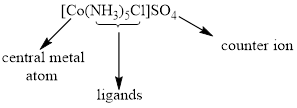Chapter 22.4, Problem 22.1CYU

Chapter
Section
Textbook Problem

(a) What is the formula of a complex ion composed of one Co3+ ion, three ammonia molecules, and three Cl− ions?(b) What is the formula for the coordination complex of an Fe2+ ion having two ethylene-diamine ligands and two bromide ion ligands?

(a)

Interpretation Introduction

Interpretation:

Formula of a complex ion composed of one Co3+ ion, three ammonia molecules and three Cl ions has to be determined.

Concept introduction:

• Coordination compounds: The compounds having coordination covalent bonds which form when metal ions react with polar molecules or anions.• Monodentate ligand: ligands which donate only one pair of electrons to form bond with metal. It only makes one bond with metal.

Examples: Cl,I

• Polydentate ligand forms two or more coordination bond with metal ions to form a complex.

Example: EDTA

• Coordination number: The total number of ligands that forms bond with the metal atom.

Coordination number of [Pt(NH3)2(Cl)2] is 4

Formulas and names of coordination compounds,

• The cation is written before the anion.
• The charge of the cation(s) is is balanced by the charge of the anion(s).
• In the complex ion, neutral ligands are written before anionic ligands and the formula for the whole is placed in a bracket.
Explanation

Given that,

Coordination complex composed of one Co3+ ion, three ammonia molecules and three Cl ions. So the net charge on the complex is zero.

The cation is Co3+, the anion is Cl</

(b)

Interpretation Introduction

Interpretation:

Formula of a coordination complex of an Fe2+ ion with two ethylene-diamine ligands and two bromide ion ligands has to be determined.

Concept introduction:

• Coordination compounds: The compounds having coordination covalent bonds which form when metal ions react with polar molecules or anions.• Monodentate ligand: ligands which donate only one pair of electrons to form bond with metal. It only makes one bond with metal.

Examples: Cl,I

• Polydentate ligand forms two or more coordination bond with metal ions to form a complex.

Example: EDTA

• Coordination number: The total number of ligands that forms bond with the metal atom.

Coordination number of [Pt(NH3)2(Cl)2] is 4

Formulas and names of coordination compounds,

• The cation is written before the anion.
• The charge of the cation(s) is is balanced by the charge of the anion(s).
• In the complex ion, neutral ligands are written before anionic ligands and the formula for the whole is placed in a bracket.

Still sussing out bartleby?

Check out a sample textbook solution.

See a sample solution

The Solution to Your Study Problems

Bartleby provides explanations to thousands of textbook problems written by our experts, many with advanced degrees!

Get Started